Ex 3.2

Chapter 3 Class 10 Pair of Linear Equations in Two Variables (Term 1)
Serial order wise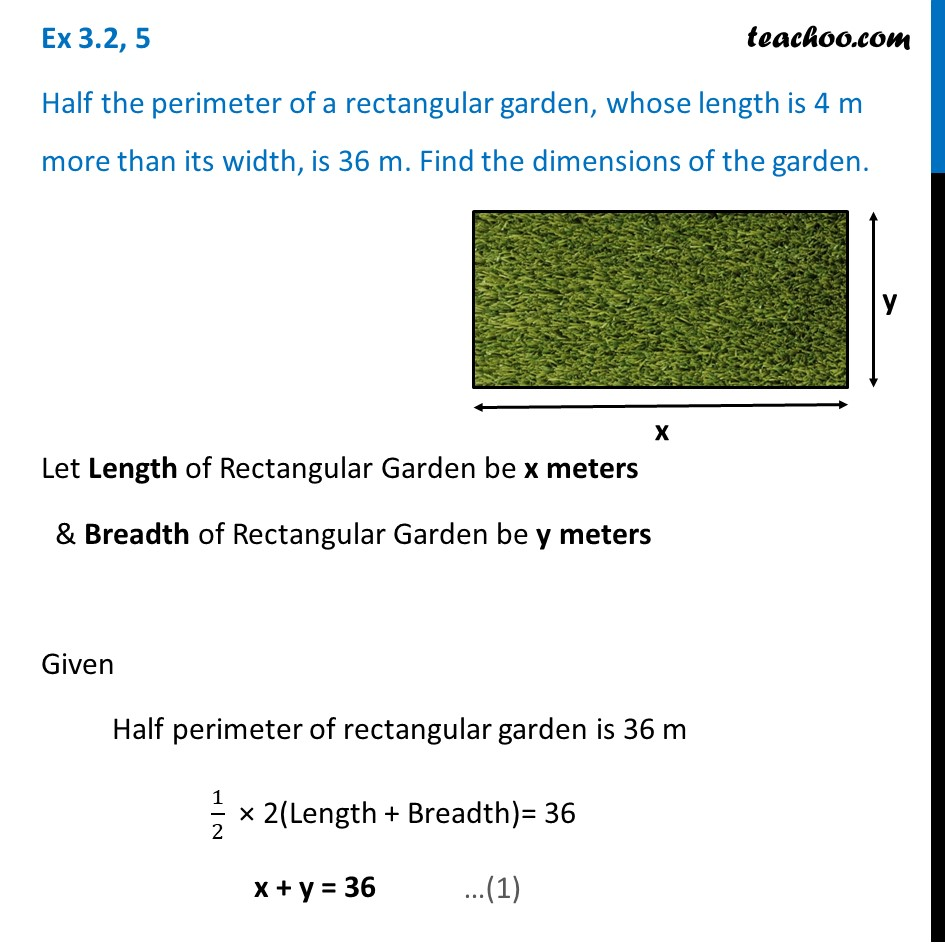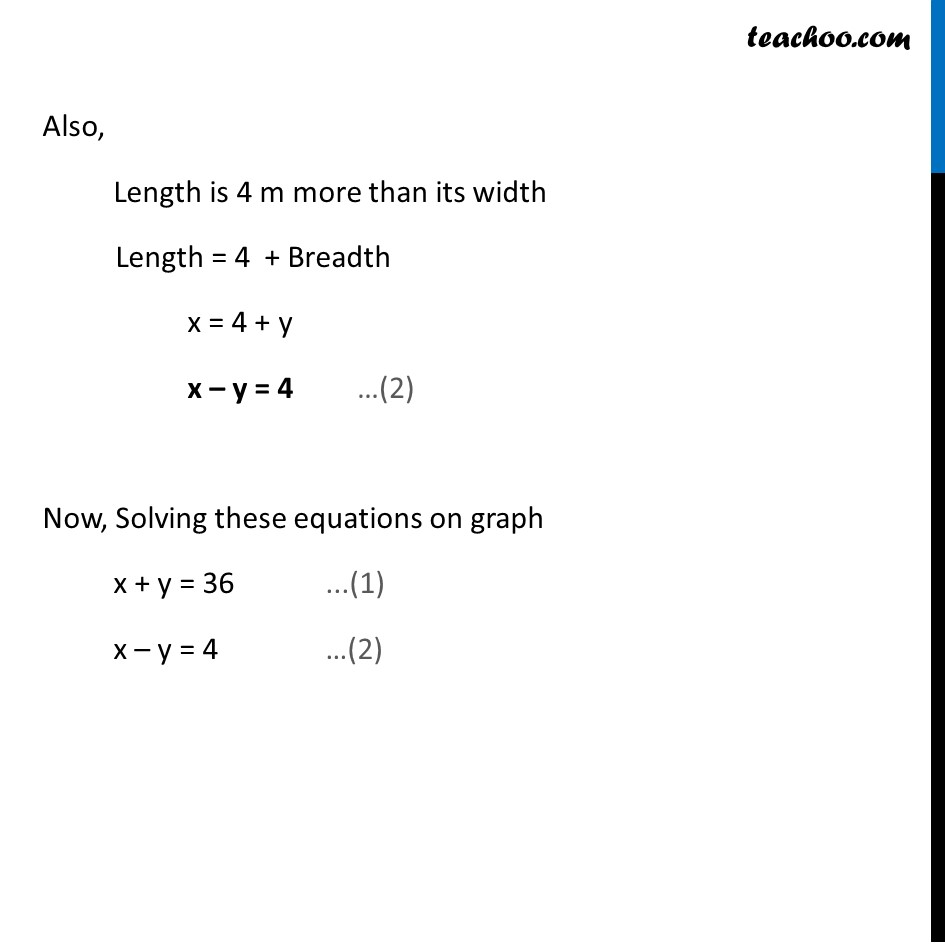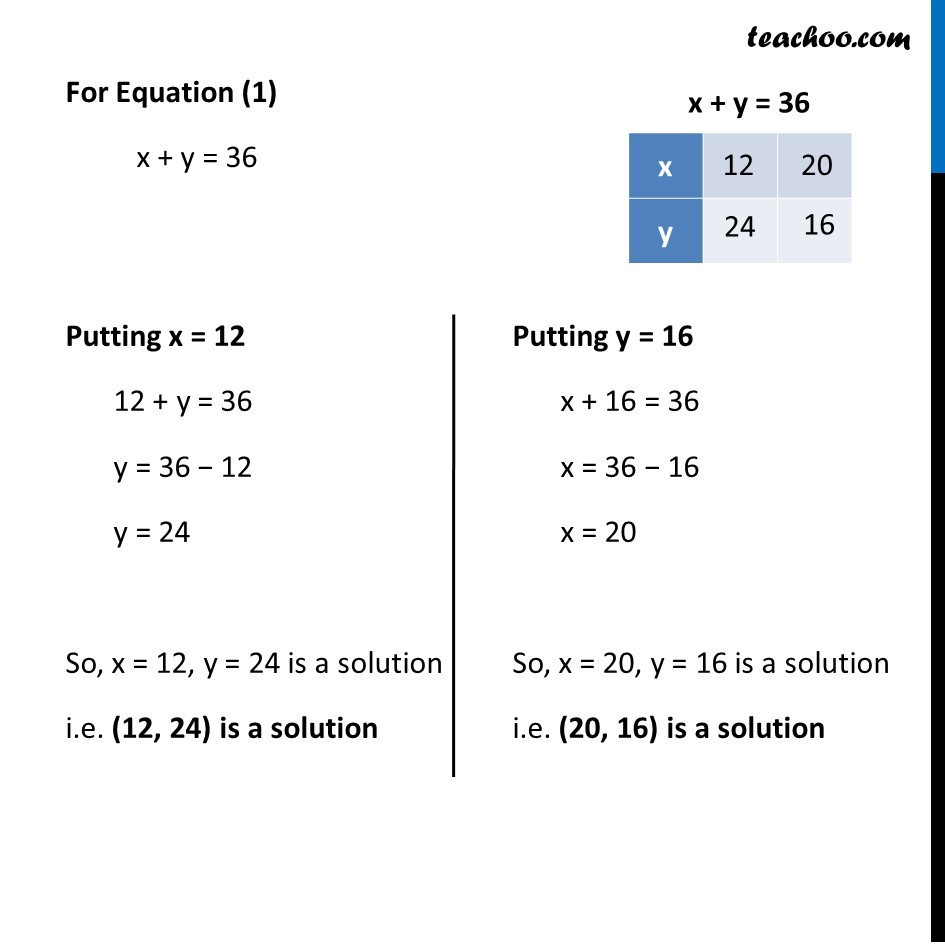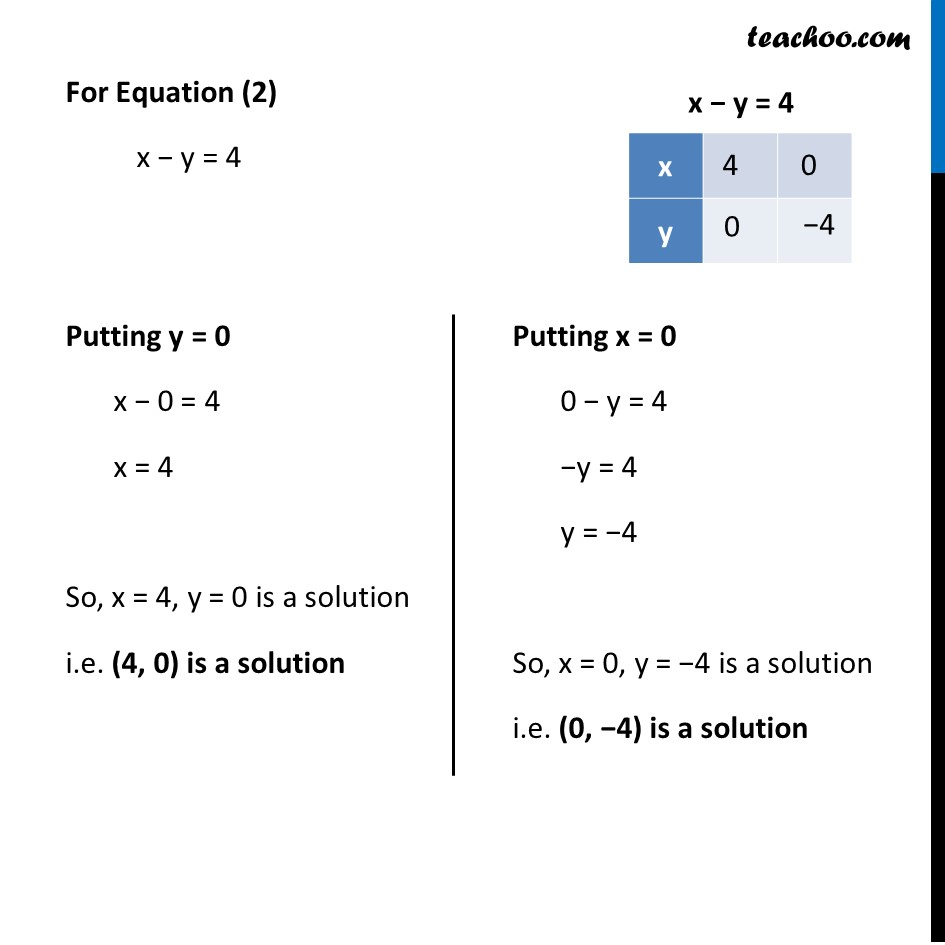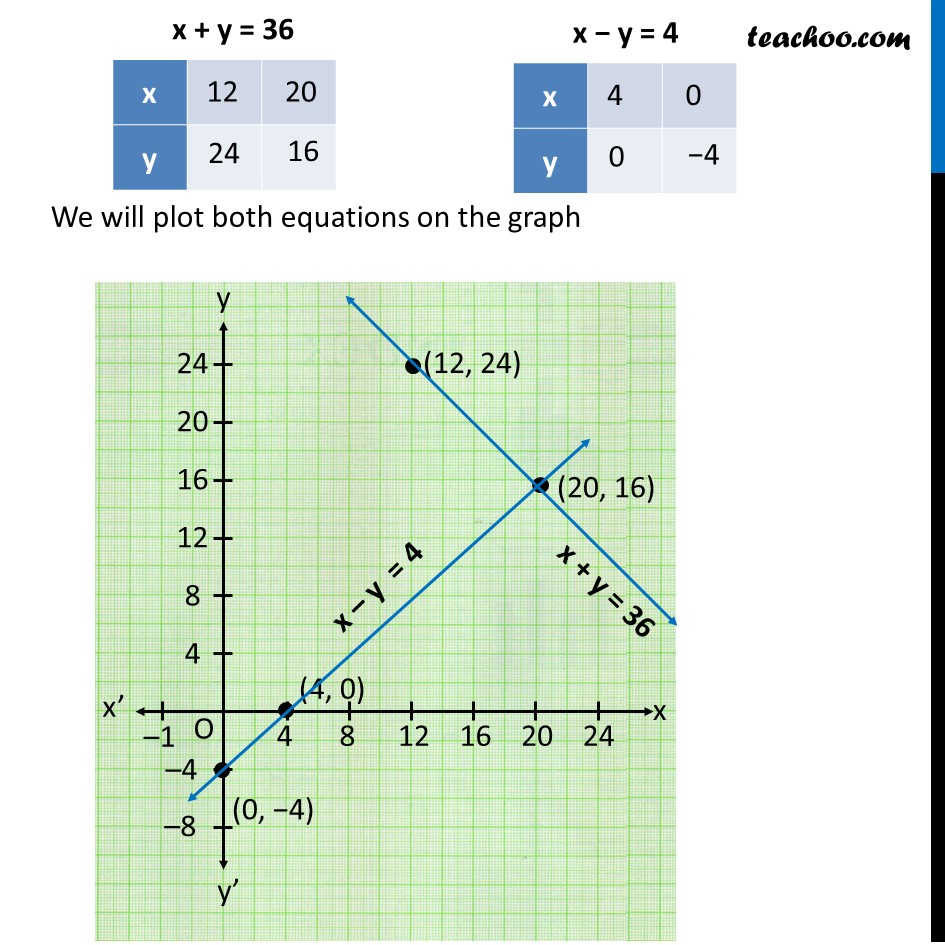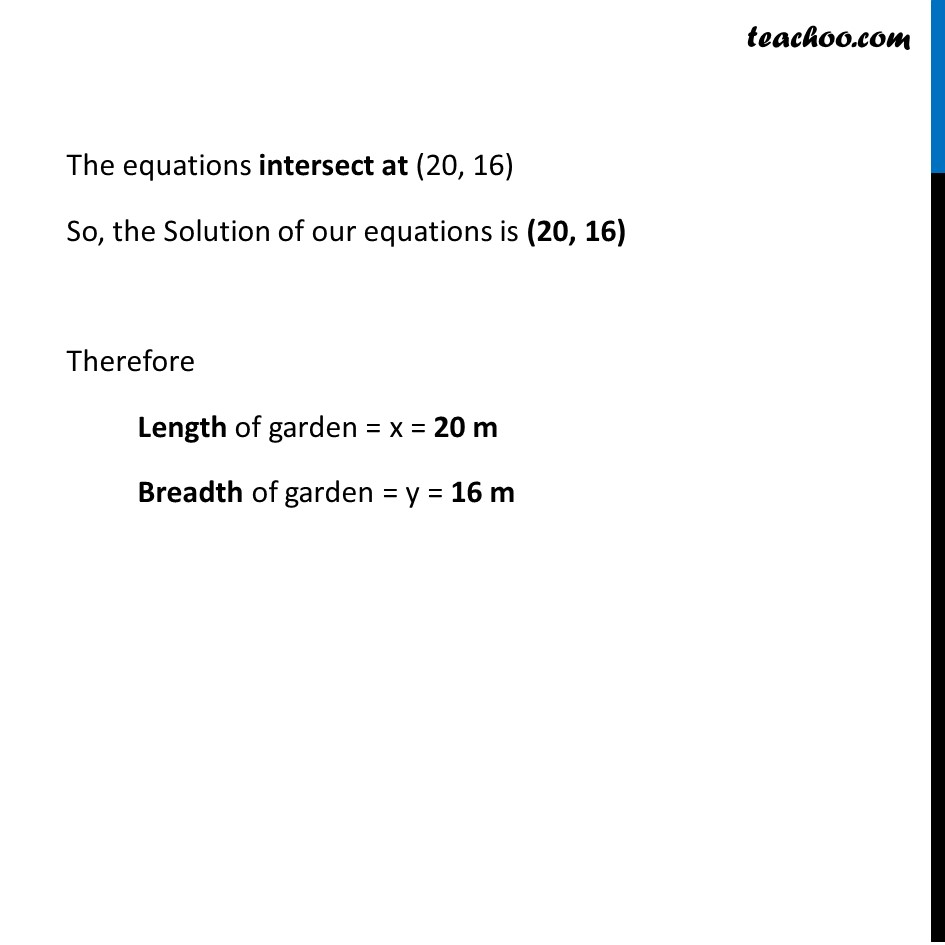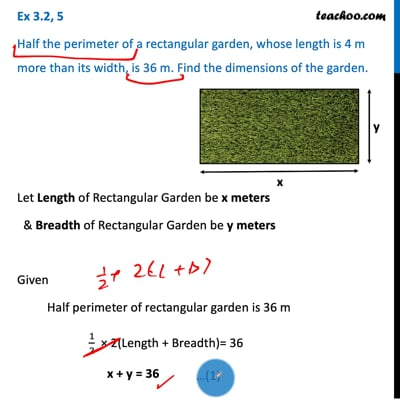This video is only available for Teachoo black users

### Transcript

Ex 3.2, 5 Half the perimeter of a rectangular garden, whose length is 4 m more than its width, is 36 m. Find the dimensions of the garden. Let Length of Rectangular Garden be x meters & Breadth of Rectangular Garden be y meters Given Half perimeter of rectangular garden is 36 m 1/2 × 2(Length + Breadth)= 36 x + y = 36 Also, Length is 4 m more than its width Length = 4 + Breadth x = 4 + y x – y = 4 Now, Solving these equations on graph x + y = 36 ...(1) x – y = 4 …(2) For Equation (1) x + y = 36 Putting x = 12 12 + y = 36 y = 36 − 12 y = 24 So, x = 12, y = 24 is a solution i.e. (12, 24) is a solution Putting y = 16 x + 16 = 36 x = 36 − 16 x = 20 So, x = 20, y = 16 is a solution i.e. (20, 16) is a solution For Equation (2) x − y = 4 Putting y = 0 x − 0 = 4 x = 4 So, x = 4, y = 0 is a solution i.e. (4, 0) is a solution Putting x = 0 0 − y = 4 −y = 4 y = −4 So, x = 0, y = −4 is a solution i.e. (0, −4) is a solution We will plot both equations on the graph The equations intersect at (20, 16) So, the Solution of our equations is (20, 16) Therefore Length of garden = x = 20 m Breadth of garden = y = 16 m